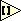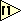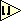# Rounding

LabVIEW has several rounding routines. They are part of the numeric palette.

The following functions are available:

## Round to nearestThis routine rounds the (floating point) input to the nearest integer. It follows IEEE854 standards for rounding the number. The IEEE standard states that if a number is exactly in between two integers (y.50) the output will be the nearest integer with an even least significant bit. This means that the number will be rounded to the nearest even integer. This approach is known under various names (banker's rounding, roundTiesToEven) and removes rounding errors in large statistical data-sets.

## Round upThis routine rounds the (floating point) input to the next bigger integer. It follows IEEE754 standards for 'Round towards' + $\infty$.

## Round downThis routine rounds the (floating point) input to the next smaller integer. It follows IEEE754 standards for 'Round towards' - $Infinity$'.